×#### Thank you for registering.

One of our academic counsellors will contact you within 1 working day.

Click to Chat

1800-1023-196

+91-120-4616500

CART 0

• 0

MY CART (5)

Use Coupon: CART20 and get 20% off on all online Study Material

ITEM
DETAILS
MRP
DISCOUNT
FINAL PRICE
Total Price: Rs.

There are no items in this cart.
Continue Shopping```Approximations

Introduction to Approximations

Approximation in Mathematics

Symbol of Approximation

Application of Derivatives for Approximation

Approximation and Errors

Linear Approximation

Formula of Linear Approximation

Approximation of pi

Approximation of pi by Various Mathematicians

Introduction to Approximations

Approximation is something which is similar to but not same as something else. The word approximation came from the  Latin word  approximates where proximus means ‘very near’ and ap means ‘to’ .Approximation is used in many fields like in mathematics, science and also in our day to day life.

Approximation in Mathematics

Approximation theory is one of the parts of mathematics. Approximation is used when it is difficult to find the exact value of any number.

It is also used to round off the errors leading to approximation.

Symbol of Approximation

Generally we use the wavy equal “≈” sign to represent the approximate values which mean “almost equal to”.

Example

π ≈ 3.14

Application of Derivatives for Approximation

We use differentiation to find the approximate values of the certain quantities. If there is a very small change in one variable correspond to the other variable then we use the differentiation to find the approximate value.

The differentiation of x is represented by dx is defined by dx = x where x is the minor change in x.

The differential of y is represented by dy is defined by dy = (dy/dx) x.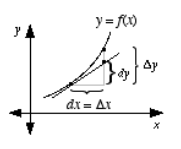As x is very small compared to x, so dy is the approximation of y.hence dy = y.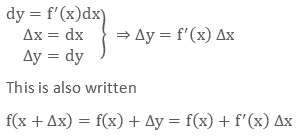This shows that the derivative of the dependent variable is not equal to the increase of the variable whereas the derivative of independent variable is equal to the increase of the variable.

Example

Find the approximate value of √26.

Solution

Here it is very easy to find the value of under root if the given number is perfect square but for such type of numbers we have to use the derivatives to find the approximate value of the function.

Let the f(x) =√x and the derivative of this is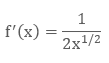Now we know the formula of approximation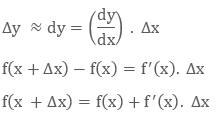Here we will assume x near to 26 which is a perfect square.

So we will assume

x = 25

x2 – x1

= 26 – 25

= 1

Here tells us the change in x.

Let x = 25 and

Now we will put the values in the formula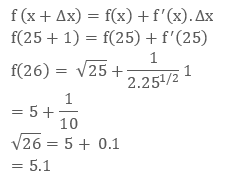Approximation and Errors

If we use the derivative of f(x) then this gives us the exact change in f(x) over the infinitely small interval dx. As we know the instantaneous rate of change which was defined using the limit as a discrete value for a change in x so that ∆x goes to zero.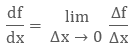If we multiply both sides by dx, we get: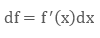We can use ∆f and ∆x  in the above equation in place of df and dx so that we get an equation that allows us to approximate the change in a function, f(x) ∆x. As we calculate the derivative at very small interval it does not give us the exact change in a function over an interval ∆x. The derivative of a function changes after each interval, dx and is constant over the interval ∆x .Therefore the following equation only represents an approximation to the net change in f(x) over a discrete interval ∆x as it assumes that the rate of change is constant.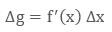Here in the below graph it is shown that as and Δx increases the approximation is getting less accurate:f(x) = x2 from x = 4 to x = 6, ∆x = 2. The change in f(x) over this interval is by definition,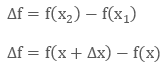By putting values in the above equationApproximate this change in f(x) by calculating the derivative at x = 4: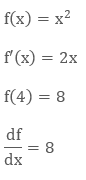By multiplying both sides by ∆x, we getBy using the derivative we got an error of 4 or 20 %. As Δx get smaller, the error gets less such that this method becomes more accurate as ∆x → dx

We can write our statement like this:If we know the value of the function at starting point f(x) then we can calculate its value at the nearest point x, by evaluating the derivative at x = a, then multiplying it by Δx = (x – a) and adding the Δf to f (a). By this we get the approximate result because it assumes the rate of change of the function is constant over the interval Δx.

But for the accurate result we should calculate f’(x) Δx over some small intervals, keeping in mind that f. (x) is different over each interval. Therefore if we multiply the derivative by Δx we get: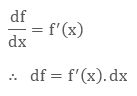The change in f is exactly equal to the derivative evaluated at a point x, multiplied by an inconsiderable dx. To get accurate approximations of f(x) we need to divide the interval into smaller sub-intervals and evaluate the rate of change at each point. For Example if we were to divide the interval from x = 4 to x = 6 of f(x) = x2  into 3 intervals to calculate the change in the functions value from x = 4 to x = 6 we would have:Direct calculations tell us that from x = 4 to x = 6, the change in f(x) is: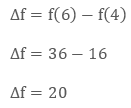From x = 4 to x = 4.67 the approximate change in f over this interval is: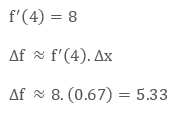From x = 4.67 to x = 5.33 the approximate change in f over this interval is: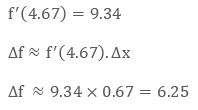Similarly, for the last interval the approximate change in f is: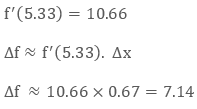Therefore, the net change in f(x) over the interval from x = 4 to x = 6 is the sum of the three-approximate change in functions that we calculated.The result corresponds to an error of just 6.6 %, far better than the 20% error we got when we used only one interval of Δx = 2.

Linear Approximation

Linear approximation is the approximation of a function using the Linear Function. It is generally used to simplify the problem to find the approximate result of the equation. In other words the linear approximation is the process to find the straight line equation,

y = mx + c, where m and c are constant.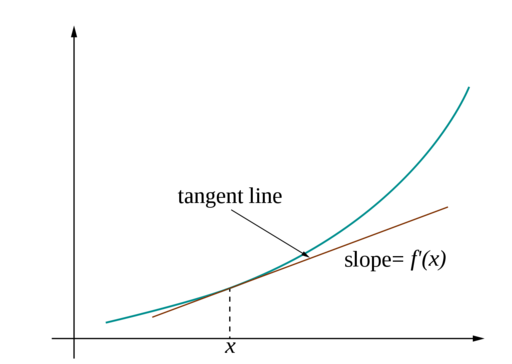Linear approximation is a powerful tool to estimate the values of a function using tangent line function. Geometrically, the secant line joining two very close points is an approximate of the tangent at one of the points.Thus the slope of the tangent line is the limit of the slope of the secant. This way the derivative of a function is interpreted as the slope of the tangent to the function at a given point. This geometrical interpretation of the derivative, gives way to estimate a function which is called Linear Approximation.

Formula of  Linear Approximation

The equation to tangent line at the point (a, f (a)) is

y = f (a) + f’ (a) (x - a).
The linear approximation to the function when x is close to a is given by
f(x) ≈ f (a) + f’ (a) (x - a)

As we use the tangent line equation for approximation, linear approximation is also called tangent line approximation. The Linear function, which represents the equation to the tangent line
L(x) = f (a) + f’ (a) (x - a) is called the Linearization of ‘f’ at ‘a’.

Linear approximation formula is therefore given by f(x) ≈ f (a) + f’ (a) (x - a)

Example 1

Estimate √4.001 by linear approximation.

Solution:

We use f(x) = √x, a = 4.

Then fꞌ (4) = 1/4,

So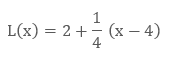This shows that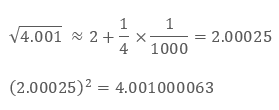Example 2

Estimate √10 using the fact that 10 = 9 + 1.

Solution:Approximation of pi

Pi is the ratio of the circumference of a circle to its diameter. This is irrational so it has an infinite amount of decimal places.

So the approximation of pi or = 3.14

Approximation of pi by Various Mathematicians

There are so many mathematicians who had given their contribution in finding the approximation of pi.

The method of Archimedes involves approximating pi by the perimeters of polygons inscribed and circumscribed about a given circle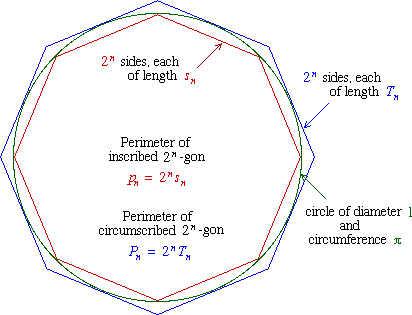In 1914, the Indian mathematician Ramanujan discovered the formula for computing Pi that converges rapidly.
In 1987, Chudnovsky brothers discovered the Ramanujan-type formula that converges more rapidly.

Ramanujan 1, 1914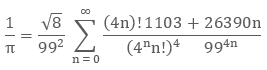Ramanujan 2, 1914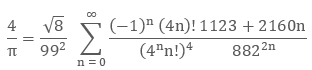Chudonovsky, 1987Approximations
```### Course Features

• 731 Video Lectures
• Revision Notes
• Previous Year Papers
• Mind Map
• Study Planner
• NCERT Solutions
• Discussion Forum
• Test paper with Video Solution